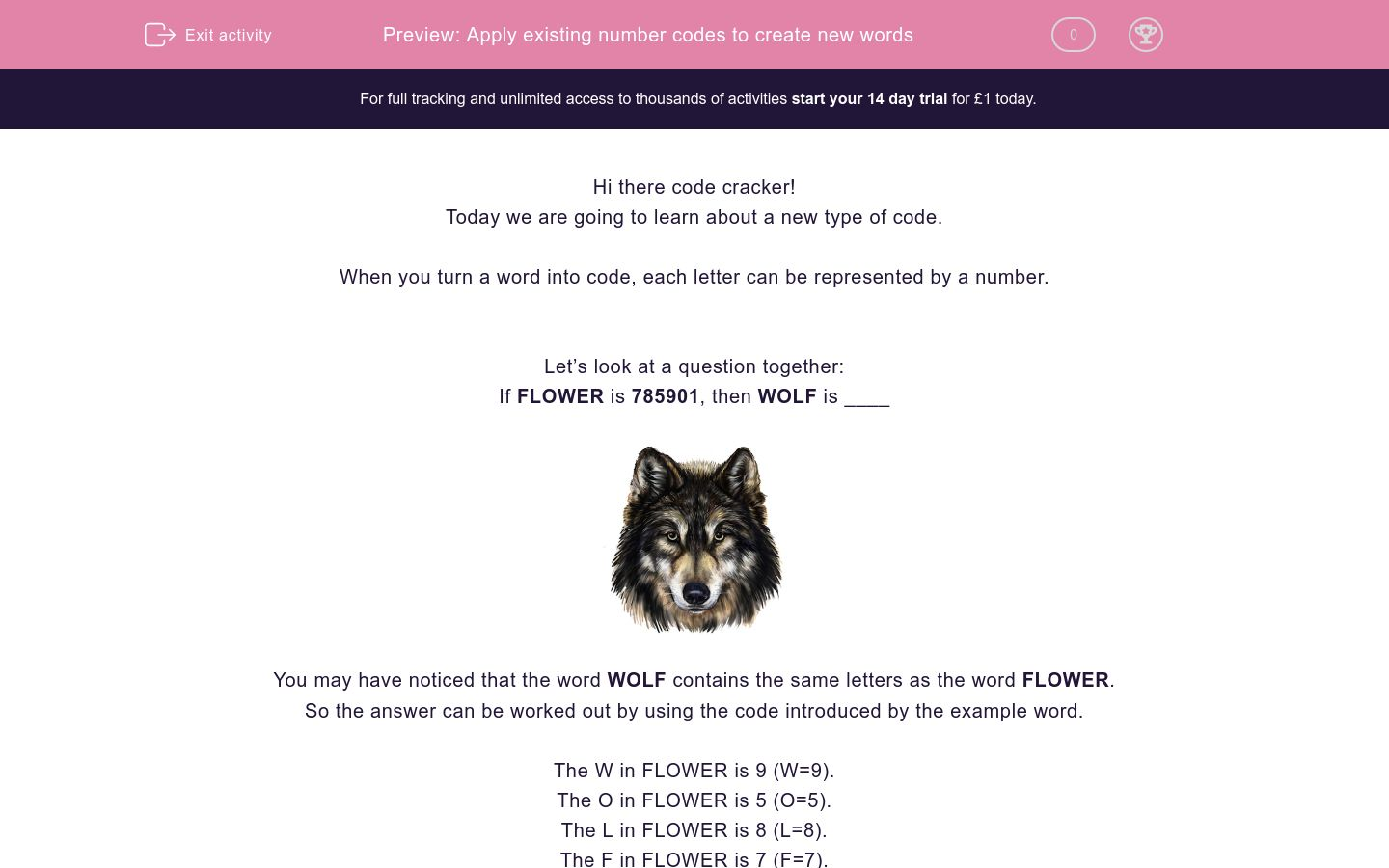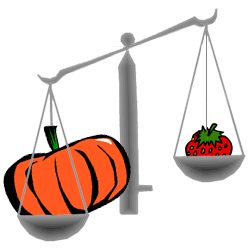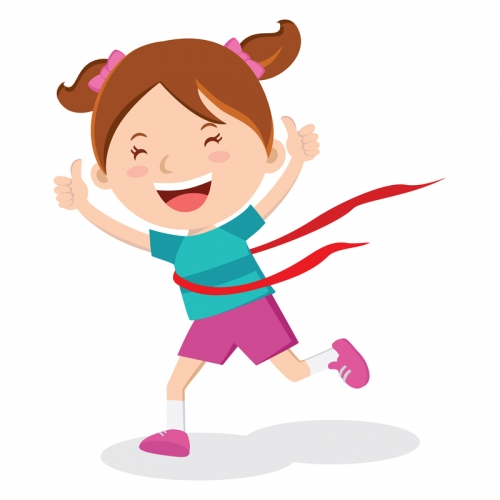# Apply existing number codes to create new words

In this worksheet, students will turn words into number codes using words with similar codes as a base. It will develop their encoding and problem solving skills.Key stage:  KS 2

Curriculum topic:   Verbal Reasoning

Curriculum subtopic:   Number Code Breaking

Difficulty level:### QUESTION 1 of 10

Hi there code cracker!

Today we are going to learn about a new type of code.

When you turn a word into code, each letter can be represented by a number.

Let’s look at a question together:

If FLOWER is 785901, then WOLF is ____You may have noticed that the word WOLF contains the same letters as the word FLOWER.

So the answer can be worked out by using the code introduced by the example word.

The W in FLOWER is 9 (W=9).

The O in FLOWER is 5 (O=5).

The L in FLOWER is 8 (L=8).

The F in FLOWER is 7 (F=7).

So WOLF is 9587 in code.

Let’s try another:

If the code for DINOSAUR is 51372894, then AROUND is ____.

The word DINOSAUR contains the same letters as AROUND, so we can use this to work out the new code.

So the code for AROUND is 847935.

In this activity, you will be a code cracker and change words into number codes. Make a note of the number that matches each letter; you may want to create your own grid to help with this.

It can be really helpful to write the word out and then write the corresponding numbers above each letter.  Give it a go!

Let’s get started!

Well hello there, code cracker! Welcome back to some more fun-filled number code breaking!

So, we are going to be trying to find the codes for words (and sometimes trying to find the codes for words, too!). Let's see how you get on...

If the code for HIPPIE is 463367, what is the code for HIP?Here's a tip for you on this one, code cracker: try and see if you can spot the shorter word contained within the longer word when changing the words into code!

If the code for GENTLEMAN is 714321690, what is the code for GENTLE?Right then, code cracker, you have done brilliantly so far! Are you getting the hang of this? Remember to keep looking for the shorter words in the longer words!

If the code for FREEDOM is 7122940, what is the code for RED?This is great work so far, code cracker! Now, we are asking you to mix up the letters a bit more in this worksheet - I know you can do it! A handy hint to help you with these questions is to write out the first word and then write the numbers of the code above the letters so that you can see which number goes with each letter.

If the code for ABSOLUTE is 93174682, what is the code for SOLE?It's Question 5, code cracker. Do you know what that means? You are officially halfway through! That is top work from you - well done! Now, let's keep at it!

If the code for IMAGINE is 3691372, what is the code for MAIN?We are definitely getting there, code cracker! We only have a few questions left so let's keep going. And remember: I'm here to help if you need me.

If the code for FUTURE is 624279, what is the code for RUT?OK, we are on to our last few questions code cracker. You've got this.

Very soon, you'll be jumping for JOY that you have finished!

If the code for ENJOY is 31629, what is the code for JOY?OK, so this is a nice short one code cracker, but don't get complacent! Make sure you take your time to work out the correct code.

If the code for POINT is 63412, what is the code for TON?We are almost at the end, code cracker! Superb work - well done! Let's take our time over the last couple of questions, though.

If the code for SURE is 9132, what is the code for USE?You made it, code breaker! What a star!Let's make sure we take care over this last question!

If the code for CURE is 9146, what is the code for CUE?• Question 1

Well hello there, code cracker! Welcome back to some more fun-filled number code breaking!

So, we are going to be trying to find the codes for words (and sometimes trying to find the codes for words, too!). Let's see how you get on...

If the code for HIPPIE is 463367, what is the code for HIP?463
EDDIE SAYS
How did you get on here, code cracker? Let's go through it, just in case you need a recap: The H in HIPPIE is 4. The I in HIPPIE is 6. The P in HIPPIE is 3. If we put it all together, we see that the code for HIP IS 463!
• Question 2

Here's a tip for you on this one, code cracker: try and see if you can spot the shorter word contained within the longer word when changing the words into code!

If the code for GENTLEMAN is 714321690, what is the code for GENTLE?714321
EDDIE SAYS
Did you spot that the word GENTLE appears in the word GENTLEMAN, code cracker? It will have really helped you to find the code if so! Let's go through it, just in case you need a recap: The G in GENTLEMAN is 7. The E in GENTLEMAN is 1. The N in GENTLEMAN is 4. The T in GENTLEMAN is 3. The L in GENTLEMAN is 2. The E in GENTLEMAN is 1. If we put it all together, we see that the code for GENTLE is 714321.
• Question 3

Right then, code cracker, you have done brilliantly so far! Are you getting the hang of this? Remember to keep looking for the shorter words in the longer words!

If the code for FREEDOM is 7122940, what is the code for RED?129
EDDIE SAYS
Did you spot that all of the letters in the word RED appear in the word FREEDOM, code cracker? It will have really helped you to find the code if so! Let's go through it, just in case you need a recap: The R in FREEDOM is 1. The E in FREEDOM is 2. The D in FREEDOM is 9. If we put it all together, we see that the code for RED is 129!
• Question 4

This is great work so far, code cracker! Now, we are asking you to mix up the letters a bit more in this worksheet - I know you can do it! A handy hint to help you with these questions is to write out the first word and then write the numbers of the code above the letters so that you can see which number goes with each letter.

If the code for ABSOLUTE is 93174682, what is the code for SOLE?1742
EDDIE SAYS
Did you use your handy hint and write out the letter and code here, code cracker? It will have really helped you if so! ABSOLUTE is quite a long word, after all. So, to find the code for SOLE, we have to work out the following: The S in ABSOLUTE is 1. The O in ABSOLUTE is 7. The L in ABSOLUTE is 4. The E in ABSOLUTE is 2. So, the code for SOLE is 1742!
• Question 5

It's Question 5, code cracker. Do you know what that means? You are officially halfway through! That is top work from you - well done! Now, let's keep at it!

If the code for IMAGINE is 3691372, what is the code for MAIN?6937
EDDIE SAYS
Do you think you are getting the hang of this, code cracker? I think you are definitely making progress - well done! Let's use our usual technique to help us. So, to find the code for MAIN, we have to work out the following: The M in IMAGINE is 6. The A in IMAGINE is 9. The I in IMAGINE is 3. The N in IMAGINE is 7. So, the code for MAIN is 6937!
• Question 6

We are definitely getting there, code cracker! We only have a few questions left so let's keep going. And remember: I'm here to help if you need me.

If the code for FUTURE is 624279, what is the code for RUT?724
EDDIE SAYS
Did you use your handy hint and write out the full word and code, code cracker? I hope so! Now, let's go through this. To find the code for RUT, we have to work out the following: The R in FUTURE is 7. The U in FUTURE is 2. The T in FUTURE is 4. So, the code for RUT is 724!
• Question 7

OK, we are on to our last few questions code cracker. You've got this.

Very soon, you'll be jumping for JOY that you have finished!

If the code for ENJOY is 31629, what is the code for JOY?629
EDDIE SAYS
Did you find this more manageable as it was a shorter word? That's quite usual if so! Also, you'll have noticed that the word JOY can be found in the word ENJOY, which makes things easier! So, to find the code for JOY: The J in ENJOY is 6. The O in ENJOY is 2. The Y in ENJOY is 9. So, the code for JOY IS 629!
• Question 8

OK, so this is a nice short one code cracker, but don't get complacent! Make sure you take your time to work out the correct code.

If the code for POINT is 63412, what is the code for TON?231
EDDIE SAYS
OK, so that should have been quite a simple one this time. We know the code for POINT, so from that we can work out the following using our usual technique: The T in POINT is 2. The O in POINT is 3. The N in POINT is 1. So the code for TON is 231!
• Question 9

We are almost at the end, code cracker! Superb work - well done! Let's take our time over the last couple of questions, though.

If the code for SURE is 9132, what is the code for USE?192
EDDIE SAYS
How was that code cracker? Short and sweet?! I hope so. We do have to clarify how we got there, though. Let's just check: The U in SURE is 1. The S in SURE is 9. The E in SURE is 2. So the code for USE is 192!
• Question 10

You made it, code breaker! What a star!Let's make sure we take care over this last question!

If the code for CURE is 9146, what is the code for CUE?916
EDDIE SAYS
OK, did we do it, code cracker?! Now, we really need to double check before you run off to eat your dinner / play in the garden / escape your 11+ practice! I don't think it's been too bad, though, has it? You've done brilliantly! So, we know the code for CURE which means we can confidently work out the code for CUE: The C in CURE is 9. The U in CURE is 1. The E in CURE is 6. So the code for CUE is 916! You made it! Wahoo!
---- OR ----